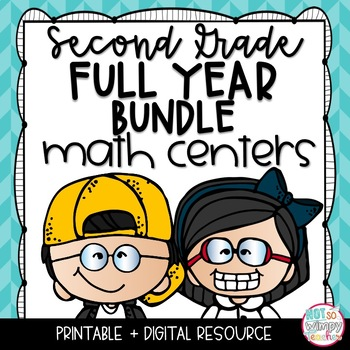# Math Centers Bundle SECOND GRADE2nd
Subjects
Standards
Resource Type
Formats Included
• Zip
Pages
400 pages
\$40.00
Bundle
List Price:
\$49.50
You Save:
\$9.50
\$40.00
Bundle
List Price:
\$49.50
You Save:
\$9.50

### Description

This 2nd grade bundle includes hands-on and engaging math centers for the entire year!!! You will be getting a total of 90 math centers!

Each set of centers has the same format, so students will learn the expectations and procedures and then be able to complete centers for the entire year without many new directions.

The centers are engaging and include sorts, task cards, math writing, color coding, matching, etc. Each set of centers has a recording book that students use for all 10 centers in that unit.

**Would you like to learn how I use these math centers? Click HERE to view a free video on how to implement math centers.**

Centers are included in color and black line for your convenience.

JUST ADDED! This bundle now includes a 40 page document with math center tips, schedules, posters, and labels!

******************************************

Centers Included:

Back to School

1. Math journaling (Subtraction)

2. Word Problems (Addition & Subtraction)

3. Roll and Build a Number (Place Value & Making Numbers)

4. Place Value (10 more and 10 less)

5. 2- Digit Addition

6. Making 10

7. Measurement (with non-standard units)

8. Missing Numbers (additions and subtraction)

9. Telling Time

10. Comparing Numbers (2-Digit)

Place Value

1. Math journaling (counting 10s)

2. Word Problems (Addition & Subtraction)

3. Pick a Number (3-Digit Place Value & Making Numbers)

4. Odds & Evens

5. Add to Subtract

6. Making 10

7. Skip Counting (counting by 1s, 2s, and 3s)

8. Number Patterns

9. Place Value Match (Expanded form and Base 10 Models)

10. Comparing Numbers (3 digit)

1. Math journaling (missing addend)

2. Word Problems (with and without regrouping, two and three addends)

3. Roll a Problem

4. Three & Four Addends

5. Using Expanded Form

6. Using Break Apart Strategy

7. Spin to Add (with and without regrouping)

8. Missing Numbers (using a hundreds chart to add)

9. Addition and Sum Matching (with and without regrouping)

10. Using a Number Line to Add

2 Digit Subtraction

1. Math journaling

2. Word Problems (with and without regrouping)

3. Roll a Problem

4. Choose a Strategy

5. Using Friendly Numbers

6. Using Break Apart Strategy

7. Draw a Word Problem (subtraction with and without regrouping)

8. Missing Numbers (using a hundreds chart to subtract)

9. Making Friendly Numbers

10. Using a Number Line to Subtract

Time and Money

1. Math journaling (adding coins)

2. Word Problems (money and change)

3. Spin a Problem (coin value)

4. Drawing clocks

5. Determining the minutes past the hour or until the next hour

6. Identifying coin value

7. Making Change

8. True or False (Does the digital time match the analog clock?)

9. Reading Analog Clocks

10. Reading clocks and AM or PM

1. Math journaling (with regrouping)

2. Word Problems (with and without regrouping)

3. Roll a Problem

4. True or False Sort (checking answers)

5. Expanded Form to Add

6. Break Apart Strategy to Add

7. Spin to Add

8. Missing Numbers in Addition Equations

9. Equation and Sum Match

10. Number Lines to Add

3-Digit Subtraction

1. Math journaling (with regrouping)

2. Word Problems (with and without regrouping)

3. Roll a Problem

4. True or False Sort (checking answers)

5. Add to Subtract

6. Break Apart Strategy to Subtract

7. Spin to Subtract

8. Missing Numbers in Subtraction Equations

9. Equation and Difference Match

10. Number Lines to Subtract

Measurement & Graphs

1. Math journaling

2. Word Problems (addition & subtraction with measurement)

3. Create a Picture Graph

4. True or False Sort (data from a bar graph)

5. Line Plot Graph

6. Picture Graph Data

7. Measure Twice (inches and centimeters)

8. Roll a Bar Graph

9. Estimate Measurement (with different units)

10. How much longer? (measuring two objects and comparing)

Geometry and Fractions

1. Math journaling

2. Drawing 2-D Figures

3. Making Shapes with Pattern Blocks

4. Naming the Fraction of a Whole

5. Making Rows and Columns

6. Attributes of Figures True/False Sort (2d and 3D)

7. Spin to Divide Shapes into Equal Shares

8. Missing Numbers (attributes of shapes)

9. Matching 2D and 3D Shapes with their Name

10. Adding Rows and Columns

Multiplication

• Equal Groups
• Skip Counting

Looking for these centers for another grade level?

Total Pages
400 pages
Included
Teaching Duration
1 Year
Report this resource to TpT
Reported resources will be reviewed by our team. Report this resource to let us know if this resource violates TpT’s content guidelines.

### Standards

to see state-specific standards (only available in the US).
Measure the length of an object by selecting and using appropriate tools such as rulers, yardsticks, meter sticks, and measuring tapes.
Measure the length of an object twice, using length units of different lengths for the two measurements; describe how the two measurements relate to the size of the unit chosen.
Estimate lengths using units of inches, feet, centimeters, and meters.
Measure to determine how much longer one object is than another, expressing the length difference in terms of a standard length unit.
Use addition and subtraction within 100 to solve word problems involving lengths that are given in the same units, e.g., by using drawings (such as drawings of rulers) and equations with a symbol for the unknown number to represent the problem.

### Questions & Answers

Teachers Pay Teachers is an online marketplace where teachers buy and sell original educational materials.